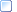﻿ Analog Data - Integer Format
 The 3D Point Data Section > Analog Data Storage

### Analog Data - Integer Format

When storing analog data using the integer C3D format, each binary sample value generated by the ADC is stored as a 16-bit integer.  By default these samples were originally stored as signed integer values although common ADC resolutions meant that all recorded values fell into the range of 0 to 32767 as positive values – negative integer sample values do not exist.

While 12-bit resolution ADC samples are common, other resolutions (i.e.,14-bit or 16-bit) may be used to store analog data. The resolution of the data may be recorded by the ANALOG:BITS parameter.  Both 12-bit and 16-bit analog sample resolutions are common although 16-bit samples may be interpreted incorrectly by applications written to read the ADC samples as signed integers.

To convert the analog sample data into physical world units, regardless of the actual sample resolution:

physical world value = (data value - offset) * channel scale * general scale

Where:

‘offset’ is in the “ANALOG:OFFSETparameters (integer)

‘channel scale’ is in the “ANALOG:SCALE” parameters (floating-point)

‘general scale’ is the “ANALOG:GEN_SCALE” parameter (floating-point)

Analog data samples are stored in a C3D file as signed integers by default although an analog to digital converter (ADC) normally generates unsigned binary values.  When generated by an ADC with up to 14-bit resolution, the sampled data can be stored within the range of values supported by the signed integer format.  For example a 12-bit ADC generates numbers in the range of 0 through 4095.  These values may be written to the C3D file as –2048 through +2047 or simply recorded as 0 through 4095.  The first range is signed (it contains both positive and negative numbers), while the second range is unsigned.  In this case, the use of signed or unsigned integers to store the analog sample is immaterial as both values fall within the range of a signed integer.  However, this is not the case when 16-bit ADC samples are stored; in this case the 16-bit data samples must be stored as signed integer numbers (the default) unless the optional parameter ANALOG:FORMAT is set to UNSIGNED.

In the absence of the ANALOG:FORMAT parameter, the format of the analog data can be determined by reading the ANALOG:OFFSET parameter.  12-bit unsigned binary values require an OFFSET of 2047 (although many programs use 2048 because their author didn’t realize that 0 is a valid number), while signed binary data will have an OFFSET of 0000.  16-bit unsigned analog data will require an OFFSET of 32767 while 16-bit signed binary data will use an OFFSET of 0000.

More:Notes for programmers - Integer Analog Data